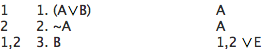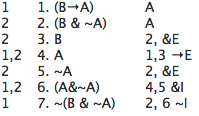# [SL13] Derivation rules 2

## §1. Rules for "v"

As for '&' and '→', there is both an elimination rule and an introduction rule for '∨':

#### ∨I (Disjunction Introduction)

If you have derived φ,
you can write down (φ∨ψ) or (ψ∨φ),
depending on everything φ depends on.

#### ∨E (Disjunction Elimination or Disjunctive Syllogism)

If you have derived (φ∨ψ) and ~ψ,
you can write down φ,
depending on everything (φ∨ψ) and ~ψ depend on.
If you have derived (φ∨ψ) and ~φ,
you can write down ψ,
depending on everything (φ∨ψ) and ~φ depend on.

The introduction rule says that you can take any formula you have written down so far and make it longer, by changing it into a disjunction. And you write down the same dependencies. So if the original formula is φ, then the new formula would be (φ∨ψ) or (ψ∨φ). Note that ψ can be any formula at all, from simple, like "A" or "B" to more complicated, like "(A & B)" or "((A→B)&(B→A))". For exampleThe elimination rule for '∨' lets you break apart a disjunction and write down only one half. Like &I, ∨E starts from two formulas and permits you to write down a third. ∨E permits you to write down φ if you have (φ∨ψ) and ~ψ in your derivation. ∨E also permits you to write down ψ, if you have (φ∨ψ) and ~φ in your derivation. The dependencies of the new formula are the same as the dependencies of the two formulas you had already. A more traditional name for this sort of rule is Disjunctive Syllogism; we will just call it ∨ Elimination, or ∨E for short.

For example:At this point you can show the interesting fact that anything is derivable from an explicit contradiction: for any φ and ψ, (φ&~φ) ⊢ ψ.

For example, (B & ~B) ⊢ A. Can you see how to show that?

Show (B & ~B) ⊢ A.

There is one more rule involving '∨', Proof by Cases, or PC:

#### PC (Proof by Cases)

If you have derived (φ∨ψ) and (φ→α) and (ψ→β),
then you can write down (α∨β),
depending on everything (φ∨ψ) and
(φ→α) and (ψ→β) depend on.

It may look complicated, but it is actually almost as simple as &I or ∨E. You need three formulas in order to apply the rule. Sometimes it may be difficult to see how to get these three formulas. But if you do have them written in your derivation, then you can write down a certain disjunction. The new formula depends on everything the three formulas you started with depend on.

Can PC be applied, starting from only 2 different formulas?

Show ((P & P) ∨ (Q & Q)) ⊢ (P ∨ Q)

Show (P ∨ P), (P → Q) ⊢ (P ∨ Q)

## §2. Rules for "~"

There are just two more rules left, the rules for the introduction and elimination of the negation symbol '~'.

Rules ~I and ~E are similar to →I in certain ways. Each of these three rules permits you to take away dependencies. In addition, with each of these three rules, you first assume something, and then you derive something, and then you apply the rule to write down a new formula.

Here is Rule ~I:

#### ~I (Negation Introduction)

If you have assumed ψ, and you have derived (φ&~φ),
then you can write down ~ψ,
depending on everything (φ&~φ) depends on except ψ.

Rule ~I provides one way to derive a negation like "~A" or "~(A&B)". Suppose, for example, you want to derive "~(A&B)". In using ~I you would first assume "(A&B)" and then derive an explicit contradiction. (An explicit contradiction is a formula of the form (φ&~φ) such as "(A&~A)" or "((B∨C)&~(B∨C))".) Then you can write down "~(A&B)".

Here is an example using ~I to show (B→A) ⊢ ~(B & ~A).In this derivation line 2 is the assumption made for the purposes of using ~I. Since we hope to show "~(B & ~A)" using ~I, we assume "(B & ~A)" and try to derive an explicit contradiction. After reaching the explicit contradiction on line 6, we can then write down on line 7 the negation of the assumption on line 2. The result on line 7 depends on everything line 6 depends on except the relevant assumption on line 2.

Notice that just a few changes turns that derivation into one that shows that (B & ~A) ⊢ ~(B→A).Show (~A∨~B) ⊢ ~(A & B) using ~I

The elimination rule for '~' is almost the same as the introduction rule. The only difference is that instead of assuming ψ and writing down ~ψ at the end, you assume ~ψ and write down ψ at the end.

#### ~E (Negation Elimination)

If you have assumed ~ψ, and you have derived (φ&~φ), then you can write down ψ, depending on everything (φ&~φ) depends on except ~ψ.

Here is an example of ~E:

~~A ⊢ A

```	1	1. ~~A			A
2 	2. ~A			A
1, 2 	3. (~A&~~A)		1, 2 &I
1	4. A			2, 3 ~E
```

You have seen all the rules of our natural deduction system! Now try making some derivations on your own.

Show the following:

1. ⊢ (~~A → A)
2. ~(P ∨ Q) ⊢ (~P & ~Q)